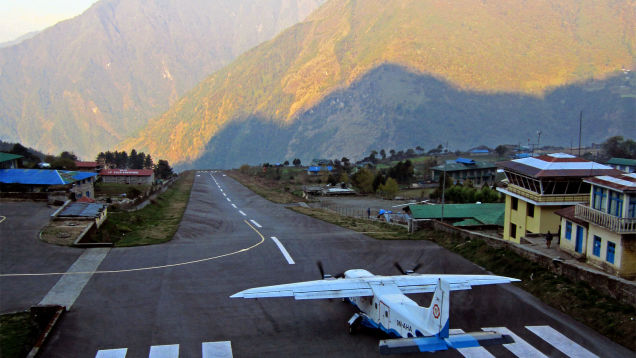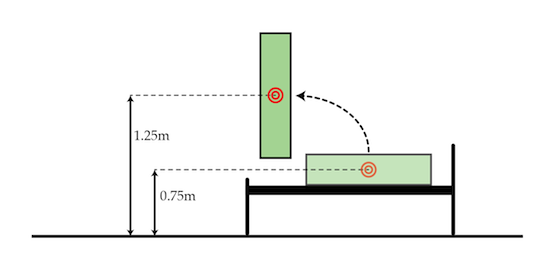Classical Mechanics

# Conservation of Energy: Level 3 ChallengesLukla airport in Nepal is one of the strangest in the world. Built to support tourism to the Himalayas, the airport has a single landing runway. What is more, the runway is only 20 m wide, 450 m long, and a 2,800 m cliff at the runway's end, leaving little room for error. In fact, the airport can only be used by so-called Short Takeoff and Landing planes (STOL). Helping somewhat is a 12% incline in the runway from start to finish, so that planes rise through over the course of their deceleration.

Suppose a STOL plane's landing speed is 45 m/s ($\approx$ 100 mph). Neglecting any other effects like wind flaps, or drag, how small will the plane's velocity (in m/s) be at the top of the runway?

Details

• The runway itself is 450 m long, i.e. if you walked from the bottom to the top, you'd walk 450 m along the runway.
• An $f$% incline means that if you walk a distance $d$ along an incline, your rise is given by $fd/100$.

Climbing out of bed is sometimes hard in the morning. If you have a mass of $60~\mbox{kg}$, what is the minimum amount of work in joules you have to do to get out of bed? Assume your center of mass when lying in your bed is $0.75~\mbox{m}$ above the floor, and your center of mass when standing is $1.25~\mbox{m}$ above the floor.Details and assumptions

• The acceleration due to gravity is $-9.8~\mbox{m/s}^2$.

Consider a system of $N$ particles whose coordinates are $\mathbf{r} = \{r_i^x, r_i^y, r_i^z\}$, and whose velocities are $\{\dot{\mathbf{r}}_i\}$.

Each pair of particles $\left(i, j\right)$ interacts through a potential $V\left(\mathbf{r}_i, \mathbf{r}_j\right)$ which has no direct dependence on time. As the system evolves in time, which of the following bulk quantities must be conserved?

A particle is constricted to move along the positive $x$-axis under the influence of a potential energy:

$U(x) = \frac{3}{x} + 7x$

Find the point of equilibrium for the particle. (Round your answer to three decimal places.)

Consider a system with $N$ particles whose coordinates are $\mathbf{r}_i = \{r_i^x,r_i^y,r_i^z\}$, and whose velocities are $\dot{\mathbf{r}}_i$. The energy of the system is described by the kinetic energy $\frac{1}{2}m\sum \dot{\mathbf{r}}^2$ and an effective potential energy term $V\left(\mathbf{r}_1, \ldots, \mathbf{r}_N\right).$

All we know about $V$ is that it depends on the positions only through their differences $\mathbf{r}_1 - \mathbf{r}_2$, i.e. $V(\{\mathbf{r}^i\}, t) = V\left(\mathbf{r}_1-\mathbf{r}_2, \mathbf{r}_1-\mathbf{r}_3, \ldots, t\right).$ As this system evolves in time, which of the following bulk quantities must be conserved?

Assumptions

• All interactions of the system with the outside world are described by $V.$
×

Problem Loading...

Note Loading...

Set Loading...